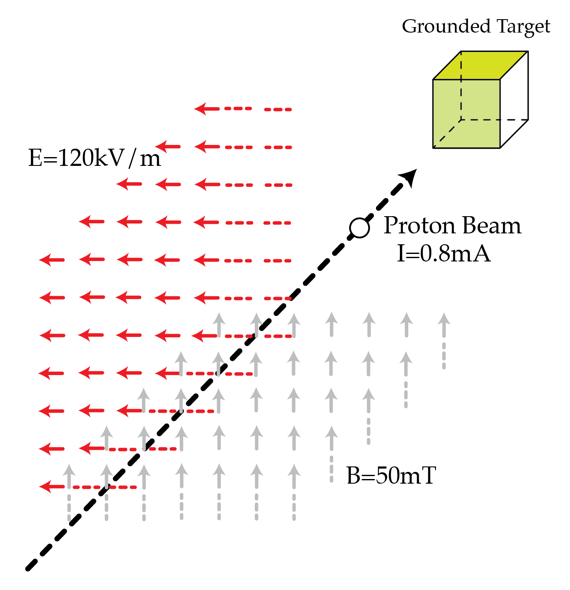# Proton Beam Hitting Its TargetA non-relativistic proton beam passes without deflection through a region where there are two transverse, mutually perpendicular, electric ($E=120 ~\textrm{kV/m}$) and magnetic ($B=50 ~\textrm{mT}$) fields. Then the beam strikes a grounded target. Find the force in Newtons with which the beam acts on the target if the beam current is $I=0.8 ~ \textrm{mA}$.

Assume that the collisions with the target are inelastic.
The proton's mass and charge are: $m_{p}=1.67 \times 10^{-27}\textrm{ kg}$ $e= 1.6 \times 10^{-19} C.$

×

Problem Loading...

Note Loading...

Set Loading...